# 【学习笔记】线段树—扫描线补充 (IC_QQQ)

(感谢 $IC$_$QQQ$ 大佬授以本内容的著作权。此人超然于世外，仅有 $Luogu$ 账号 尚可膜拜)

【学习笔记】线段树详解（全）

## 上题：

    给出N个矩形，求最后所得的图形的面积（周长）。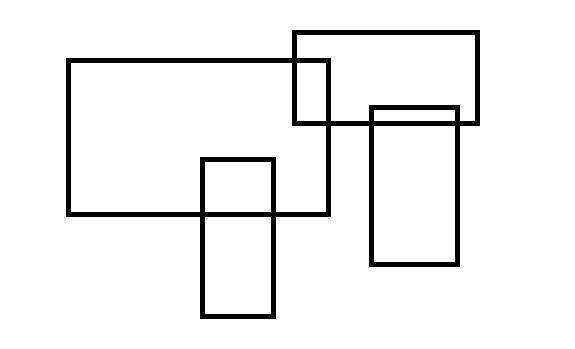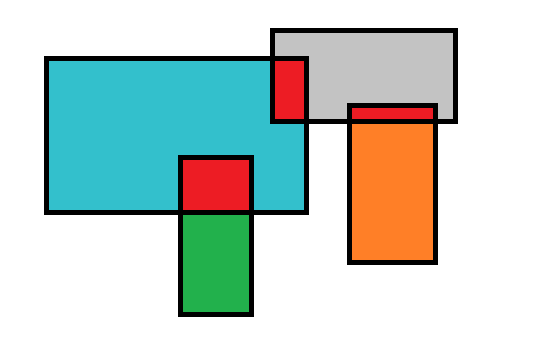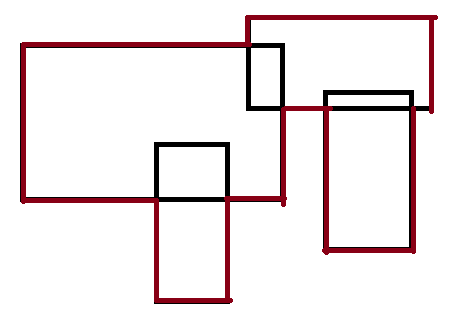## 先解决简单一些的面积：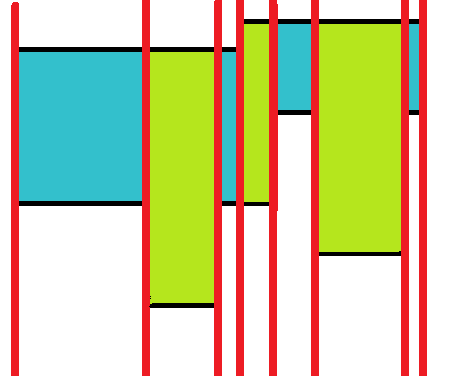## 下一步：

• 这条扫描线到达了边$x$,修改扫描线。
• 扫描线的状态固定了，影响一直持续到下一条边$x’$
• 我们找到扫描线被覆盖的部分长度和$H$
• 那这一部分（$x$$x’$）的面积就找到了：$H*(x’-x)$$ans$加上这一部分。

$emmmmm$，可以看到，这个流程并不好走，我们遇到了一抹多的问题。

## H怎么找

$i$段的长度就是$row[i+1]-row[i]$

void pre_work_row(){
sort(row+1,row+1+2*n);//排序
tot=unique(row+1,row+1+2*n)-(row+1);//去重
return;
}
int query(int val){//查询代表数
return lower_bound(row+1,row+1+tot,val)-row;
}


## 【题目链接】

$Atlantis$ $[POJ1151]$

【标签】线段树/扫描线

【标签】线段树/扫描线

## 【题解】Atlantis

### 描述

样例输入：
2
10 10 20 20
15 15 25 25.5
0

Test case #1
Total explored area: 180.00


### 代码实现：

#include<cstdio>//一定在VJ上选C++
#include<cstring>
#include<iostream>
#include<algorithm>
using namespace std;
const int N=205;
int n,nn,tot,Case;//n:矩形个数，nn:边的数量（2*n）
//tot:离散化后，代表数的个数
double ans,row[N];//答案，离散化数组
struct node{
int k;double x,u,v;//横坐标，纵坐标，v>u
}da[N];//四元组
struct aaa{int l,r,cnt;double len;}tree[4*N];//数组大小是重点
bool cmp(node a,node b){return a.x<b.x;}
void pre_reset(){
nn=2*n;ans=0;tot=0;
memset(da,0,sizeof(da));
memset(row,0,sizeof(row));
memset(tree,0,sizeof(tree));
}
void scan_work(){
double a,b,c,d;
for(int i=1;i<=n;i++){
scanf("%lf%lf%lf%lf",&a,&b,&c,&d);
row[i]=b;row[i+n]=d;
da[i].x=a;da[i+n].x=c;
da[i].u=b;da[i+n].u=b;
da[i].v=d;da[i+n].v=d;
da[i].k=1;da[i+n].k=-1;
}
sort(da+1,da+1+nn,cmp);
return;
}
void pre_row_work(){
sort(row+1,row+1+nn);
tot=unique(row+1,row+1+nn)-(row+1);
return;
}
int query(double val){
return lower_bound(row+1,row+1+tot,val)-row;
}
void built_work(int pos,int l,int r){
tree[pos].l=l;tree[pos].r=r;
if(l==r) return;
int mid=(l+r)>>1;
built_work(pos*2,l,mid);
built_work(pos*2+1,mid+1,r);
return;
}
void push_up(int pos){
int ls=pos*2,rs=pos*2+1;
if(tree[pos].cnt)//如果当前区间被覆盖
tree[pos].len=row[tree[pos].r+1]-row[tree[pos].l];
else{//当前区间没有被覆盖
if(tree[pos].l==tree[pos].r) tree[pos].len=0;//只有一段
//也可以不加这个特判，但tree要再开大一倍，防止越界
else tree[pos].len=tree[ls].len+tree[rs].len;
}
return;
}
void change(int pos,int l,int r,int w){
if(l<=tree[pos].l&&r>=tree[pos].r){
tree[pos].cnt+=w;
push_up(pos);//传递信息
return;
}
int mid=(tree[pos].l+tree[pos].r)>>1;
if(l<=mid) change(pos*2,l,r,w);
if(r>mid) change(pos*2+1,l,r,w);
push_up(pos);//传递信息
return;
}
int main(){
while(1){
scanf("%d",&n);
if(n==0) break;
pre_reset();//清零
scan_work();//读入
pre_row_work();//离散化
built_work(1,1,tot-1);//建树
for(int i=1;i<nn;i++){
int u=query(da[i].u);
int v=query(da[i].v)-1;
change(1,u,v,da[i].k);//修改扫描线
double H=tree.len;
ans+=(da[i+1].x-da[i].x)*H;
}
printf("Test case #%d\n",++Case);
printf("Total explored area: %.2lf\n\n",ans);
}
}


## 【题解】矩形周长 Picture

### 举例说明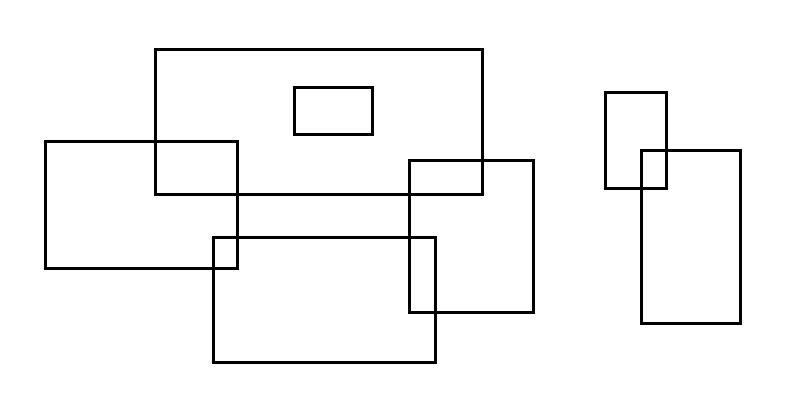### 尝试解决：

$x$为关键元从小到大排序这些四元组。

### 然后呢？然后怎么办：

• 修改扫描线。
• 查询扫描线被覆盖长度$len$

$ans$累加上高和长就好了。

### 用线段树优化：### 代码实现：

#include<cstdio>
#include<cstring>
#include<iostream>
#include<algorithm>
using namespace std;
const int N=1e4+5;
int n,nn,ans;
int tot,last;//last：上次扫描线被覆盖长度
int row[N];
struct node{int x,u,v,k;}da[N];
struct aaa{
int cnt,num,len;
bool lg,rg;
}tree[4*N];
bool cmp(node a,node b){
if(a.x!=b.x) return a.x<b.x;
return a.k>b.k;
}
void pre_work_row(){
sort(row+1,row+1+nn);
tot=unique(row+1,row+1+nn)-(row+1);
return;
}

int query(int x){
return lower_bound(row+1,row+1+tot,x)-row;
}
void Pushup(int pos,int pl,int pr){
int ls=pos*2,rs=pos*2+1;
if(tree[pos].cnt){
tree[pos].num=1;
tree[pos].len=row[pr+1]-row[pl];
tree[pos].lg=tree[pos].rg=1;
}
else{
tree[pos].num=tree[ls].num+tree[rs].num;
if(tree[ls].rg&&tree[rs].lg) tree[pos].num--;
tree[pos].len=tree[ls].len+tree[rs].len;
tree[pos].lg=tree[ls].lg;
tree[pos].rg=tree[rs].rg;
}
return;
}
void change(int pos,int pl,int pr,int l,int r,int w){
if(l<=pl&&r>=pr){
tree[pos].cnt+=w;
Pushup(pos,pl,pr);
return;
}
int mid=(pl+pr)>>1;
if(l<=mid) change(pos*2,pl,mid,l,r,w);
if(r>mid) change(pos*2+1,mid+1,pr,l,r,w);
Pushup(pos,pl,pr);
return;
}
int main(){
scanf("%d",&n);
int a,b,c,d;nn=2*n;
for(int i=1;i<=n;i++){
scanf("%d%d%d%d",&a,&b,&c,&d);
row[i]=b;row[i+n]=d;
da[i].x=a;da[i+n].x=c;
da[i].u=b;da[i+n].u=b;
da[i].v=d;da[i+n].v=d;
da[i].k=1;da[i+n].k=-1;
}
pre_work_row();
sort(da+1,da+1+nn,cmp);
for(int i=1;i<=nn;i++){
last=tree.len;
change(1,1,tot-1,query(da[i].u),query(da[i].v)-1,da[i].k);
ans+=abs(tree.len-last);
ans+=(da[i+1].x-da[i].x)*tree.num*2;
}
printf("%d",ans);
return 0;
}

posted @ 2019-06-03 13:10  辰星凌  阅读(414)  评论(0编辑  收藏  举报